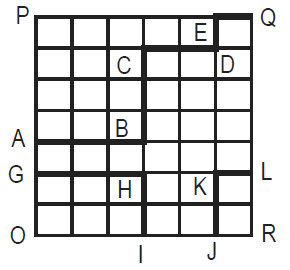Question 8

#What is the area under the line GHI-JKL in the given quadrilateral OPQR, knowing that all the small spaces are squares of the same area? I. Length ABCDEQ is greater than or equal to 60. II. Area OPQR is less than or equal to 1512.

Solution

Let the side of the smallest square be x.

According to statement 1,$$10x\geqslant 60$$

$$x\geqslant 6$$

According to statement 2, $$7x*6x\leq 1512$$

$$x\leq 6$$

From both the statements x = 6 and the answer can be determined.